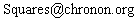## Pyramid Puzzle

It is known that
 24 Σ n=1
n2 = 4900 = 702
and that this is the only integer solution to
 N Σ n=1
n2 = M2
(apart from the trivial N=1=M). This means that if you had a square pyramid with 24 layers made of stacked balls, then they could be rearranged to form a 70 × 70 square.

But supposing the balls in each layer were stuck together. Would it be possible to arrange these 24 squares to make the 70 × 70 square. The following applet has been written to test this out.

 You do not appear to have Java enabled on this browser. (Note that Java is no longer supplied by default on Windows systems). The latest Java environment can be downloaded and installed from the Java website
Each square can be dragged using the mouse and dropped into position on the 70 × 70 grid. A square can only be dropped into space that is not already occupied, otherwise it will jump back to its position outside the grid. If a square can be dropped then the cursor will show as a hand.

Be warned that the puzzle is almost certainly impossible. Indeed, examples of fitting any set of squares together to make a larger square are hard to find. But I thought it was worth writing this applet to see what could be done. Even if the full puzzle is impossible, it might be interesting to see whether it is possible to make a smaller square using some of the pieces available. If you do this then do let me know at :I would also be interested to hear about any proofs of the impossibility of the puzzle.

If you have 4900 balls to spare then you might like to try making a physical version of this puzzle, although I guess it would take up quite a bit of space.

Note: Apparently the puzzle was in fact been shown to be impossible in 1975. Further details are available at http://home.datacomm.ch/t_wolf/tw/misc/squares.html